### Defining the Terms

Acid: A non-metal oxide, which when dissolved in water, releases hydrogen
ions H+.

Alkali: The oxide of an alkali metal or alkaline earth metal, which when dissolved in water, releases hydroxide
ions OH-.

Amphoteric: Able to act either as an acid or as a base/alkali.

Atomic Weight: The mass of an atom of a chemical element expressed in atomic mass units. It is approximately equivalent to the number of protons and neutrons in the atom (the mass number).

Molecular Weight: The ratio of the average mass of one molecule of an element or compound to one twelfth of the mass of an atom of carbon-12. For example, the molecular weight of water, which has two atoms of hydrogen and one atom of oxygen, is 18 (i.e., 2 + 16).

Unity Molecular Formula (UMF)—also known as a Seger Formula: Glaze recipe format based on the number of molecules instead of on weights of raw materials, where the total molecules of flux in a glaze are calculated to total 1.0 (which means they are in unity).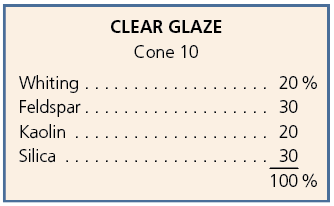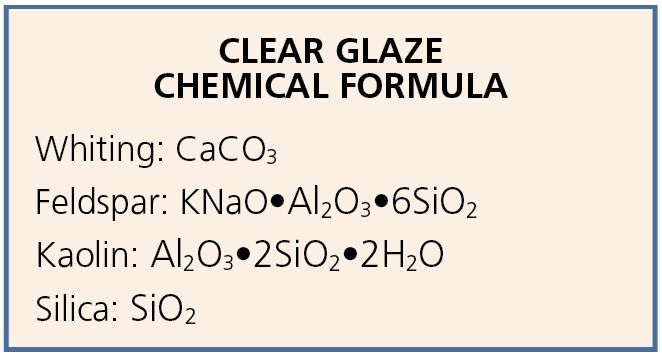### Glaze Formulas

A glaze recipe can be reduced to a chemical formula. This makes it easier to compare with other glazes or to substitute different materials to obtain the same glaze recipe. There are many software programs that take care of glaze-formula calculation; however, it is useful to have an understanding of how glaze formulas are calculated and to be able to work out glaze material substitutions for your own glazes.

A glaze recipe shows the amount of each material by weight percent. This type of recipe is easy to read and is used for mixing a glaze batch from raw materials (1). Additionally, each material in the recipe has a chemical formula (2).

The glaze formula is the sum total of all the oxides, in the same molecular proportions given in the glaze recipe (i.e. the recipe weight divided by the molecular weight). The water, H2O, and carbon dioxide, CO2, are given off in the firing. The glaze formula (0.2K2O, 0.1NaO, 0.7CaO)•0.5Al2O3•4.0SiO2 adds together the molecules in the recipe (note: the fluxes add up to 1 as they do in figure 3). In this example, the formula is very similar to the formula for feldspar or Cornwall Stone, which is almost a glaze in itself if fired to a high temperature.

The table below shows a more precise version of this glaze formula, known as the Seger unity formula or Unity Molecular Formula (UMF), which shows the exact number of molecules of each flux, silica, and alumina (3). This is very accurate, but not the easiest notation to use in practical glaze development. The oxides are arranged in three columns: alkaline, amphoteric, and acidic. In the unity formula, the total of all the alkaline fluxes is made to add up to 1. This is almost the same as the simplified glaze formula in figure 2, but the quantities have been adjusted so that all the fluxes (K, Na, and Ca) add up to 1 molecule, so instead of 1 molecule of alumina and 8 silica, there are 0.5 alumina and 4 silica molecules. This enables direct comparison between glazes, regardless of the particular materials used in the recipe. The ratio of alumina to silica tells us something about the glaze properties. A ratio of 1:5 would give a matte glaze and a ratio of 1:8 gives a glossy glaze. In between matte and glossy are satin mattes, becoming gradually more glossy.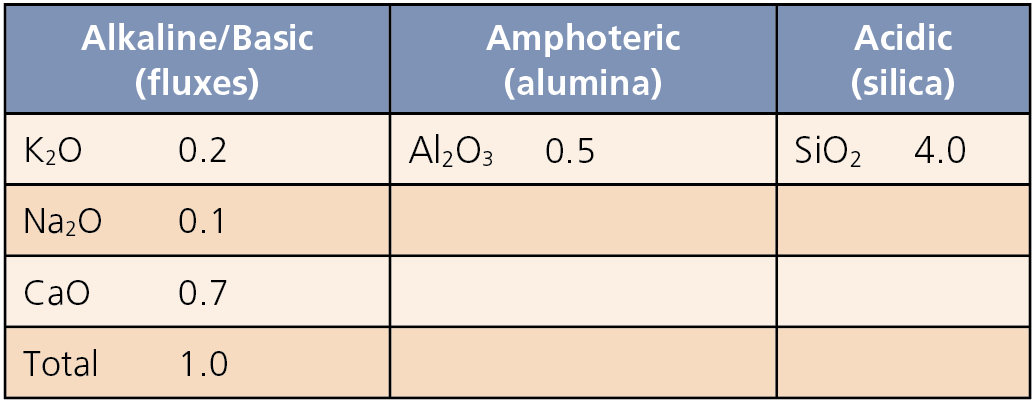### How to Calculate Glaze Material Substitution

Similar glaze materials can be substituted in a glaze recipe, for example, barium carbonate can be substituted by strontium carbonate, which is not toxic. To calculate the weight of material needed to give the same number of molecules, the recipe weight should be divided by the molecular weight. Barium carbonate has a molecular weight of 197.3, while strontium carbonate has a molecular weight of 147.6 (obtained by adding the atomic weights of strontium, carbon, and 3 oxygen atoms, from the chemical formula SrCO3). The atomic weight is the combined weight of protons and neutrons. As different isotopes have different numbers of neutrons, the weight is averaged, which explains why the atomic weights are not whole numbers. Since a strontium carbonate molecule weighs less than a barium carbonate molecule, less strontium carbonate (by weight) will be needed to substitute for the barium to maintain the same molecular ratio. Using the molecular weights above, dividing 147.6 by 197.3 gives 0.75, so the weight of strontium carbonate required to give the same number of molecules in the glaze is 0.75 times the weight of barium carbonate. A similar calculation can be made, for example, to find the amount of cobalt carbonate required to replace cobalt oxide in a recipe (in this case, around 1.5 times).

Some substitutions are more complicated. Whiting (CaCO3) can be replaced by wollastonite (CaSiO3), but the same number of molecules of silica must be subtracted from the total amount of silica in the glaze formula, as wollastonite contains both calcium and silica.

Other substitutions are very simple, for example, various feldspars are interchangeable weight for weight, although the glaze may change in character, depending on the composition of the feldspar. Naturally occurring minerals do not always have precise chemical formulas, but contain impurities that affect the material’s properties. It is useful to know which glaze materials can be substituted for others (4), as you may not have all the materials required in a particular recipe. If you are new to glaze mixing, it is only necessary to buy a few essential materials at first, including feldspar, whiting, silica, and kaolin. If you are planning to make mid-range glazes, a borate frit such as Ferro frit 3134 will also be useful. Earthenware and raku glazes consist mostly of frit, with some clay and silica.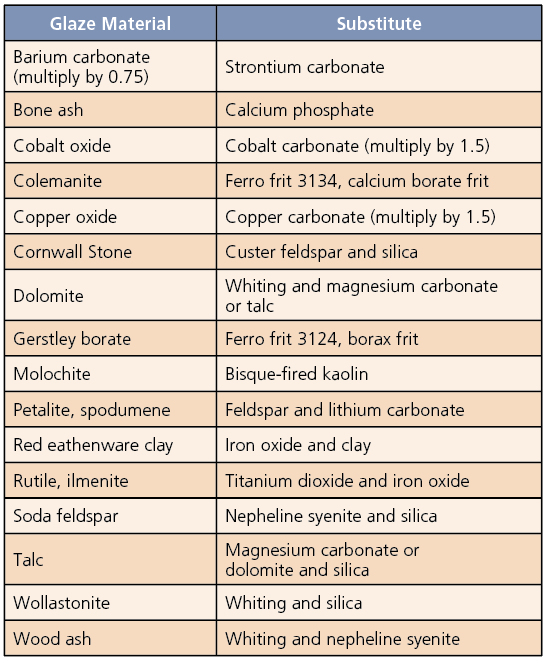### What Makes a Stable Glaze

Through trial and error, a set of limits has been determined, particularly for the amounts of silica and alumina in a glaze. Inside these limits, the glaze will be stable, insoluble, and reasonably hard-wearing. It will be resistant to attack by acids in foods and alkalis in the dishwasher. However, many interesting glazes are outside these limits, such as copper-red glazes, which have high alkali and low alumina. Matte glazes are likely to have lower resistance to attack by acids than shiny glazes. This is because matte surfaces are made up of microscopic crystals, which present more surface area for reaction with acids.

### Hardness and Scratch Resistance

In general, it is better to use a mixture of several different fluxes than to rely on only one or two. Potash feldspar will make harder glazes with better scratch resistance than soda feldspar. The alkaline earths (calcium, magnesium, barium, and strontium) will increase glaze stability, as will zinc oxide. Adding titanium or rutile will also help to improve resistance to acid attack. Adding zirconium silicate makes the glaze opaque and also improves durability and scratch resistance. Low-alumina glazes may become scratched more easily than those within the limits for stability. In general, the higher the firing temperature, the harder and more durable the glaze will be, provided it contains enough alumina and silica. For earthenware and mid-range glazes, enough boron must be added to allow complete melting at the chosen firing temperature.

Matte and crystalline glazes may be marked by cutlery. This occurs when metal from the cutlery scrapes across and is deposited on the microscopically rough edges of crystals in the glaze. It is better not to use matte glazes on surfaces of functional pots that will come into contact with cutlery, although most marks can usually be removed. Zirconium-opacified glazes may also by marked by cutlery, however, these marks may not be removable.

### Limits for Stable Glazes

To find out whether a glaze is within the limits for stability (5, 6), its unity formula must first be calculated. Though this can be done manually, the fastest way to do this is to use available glaze calculation software programs. The recommended amounts of alumina and silica in glazes increase with firing temperature. Glazes within these limits will be glossy glazes. Matte and crystalline glazes will often be outside these limits.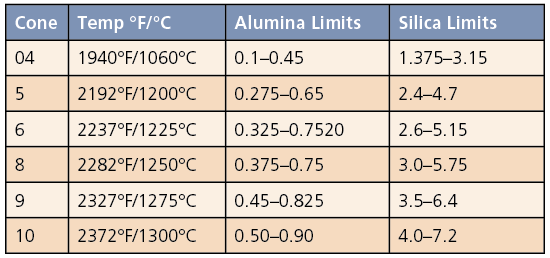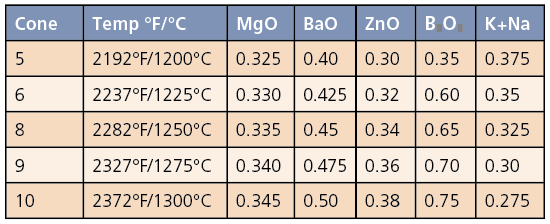the author Excerpted from Science for Potters by Linda Bloomfield. Published by The American Ceramic Society and available in the Ceramic Arts Network shop (https://mycan.ceramicartsnetwork.org).

Topics: Glaze Chemistry